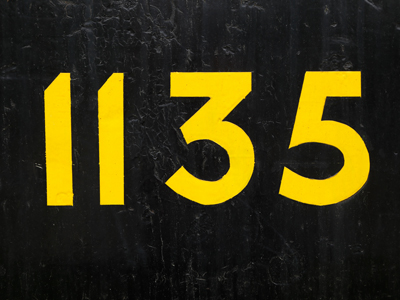Three is in the tens' place in the number 1,135.

# Place Value 2 (Easy)

If you’ve already played our first set of Eleven-Plus maths quizzes on Place Value (the Very Easy ones), then you’ll know that the values of digits within longer numbers change depending on their position. This second, and slightly more difficult, set of quizzes tests you on numbers between 10,000 and 99,999.

Unlike reading words, you work out the value of a digit within a longer number by reading from right to left – NOT left to right. Their values go like this:

• First number – Ones
• Second number – Tens
• Third number – Hundreds
• Fourth number – Thousands
• Fifth number – Ten thousands

Here’s an example: in the number 98,765 the 5 is ones, the 6 is tens, the 7 is hundreds, the 8 is thousands and the 9 is ten thousands. It’s really easy once you’ve got the hang of it!

But have you got the hang of it? Try this quiz to find out. Can you get all the questions right?

1.
What is the digit in the tens' place in 80,926?
0
9
2
6
Reading the number from right to left: 2 is in the tens' place
2.
What is the place value of 4 in 84,726?
The ten thousands' place
The thousands' place
The hundreds' place
The tens' place
Reading the number from right to left: 4 is in the thousands' place
3.
What is the digit in the thousands' place in 68,427?
6
8
4
2
Reading the number from right to left: 8 is in the thousands' place
4.
What is the digit in the ten thousands' place in 93,745?
9
3
7
4
Reading the number from right to left: 9 is in the ten thousands' place
5.
What is the place value of 7 in 17,416?
The tens' place
The hundreds' place
The thousands' place
The ten thousands' place
Reading the number from right to left: 7 is in the thousands' place
6.
What is the digit in the ones' place in 65,902?
6
5
0
2
Reading the number from right to left: 2 is in the ones' place
7.
What is the place value of 7 in 89,473?
The tens' place
The hundreds' place
The thousands' place
The ten thousands' place
Reading the number from right to left: 7 is in the tens’ place
8.
What is the place value of 2 in 27,601?
The tens' place
The hundreds' place
The thousands' place
The ten thousands' place
Reading the number from right to left: 2 is in the ten thousands' place
9.
What is the place value of 9 in 17,985?
The ones' place
The tens' place
The hundreds' place
The thousands' place
Reading the number from right to left: 9 is in the hundreds' place
10.
What is the digit in the hundreds' place in 53,791?
5
3
7
9
Reading the number from right to left: 7 is in the hundreds' place
Author:  Frank Evans